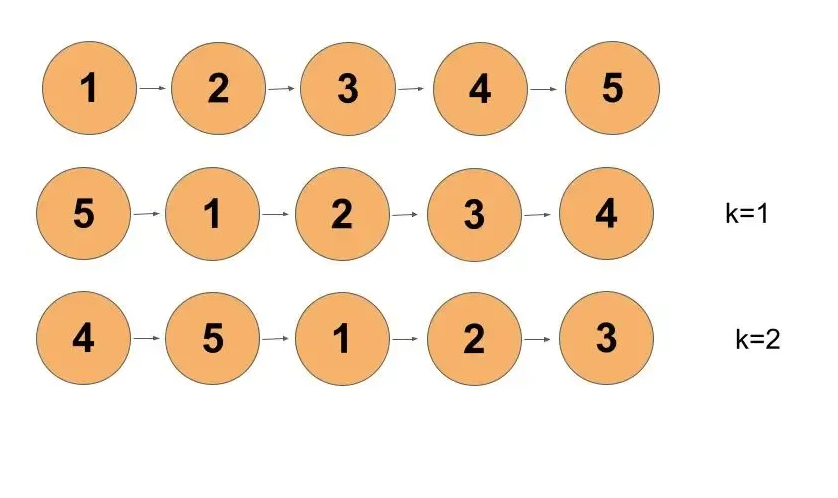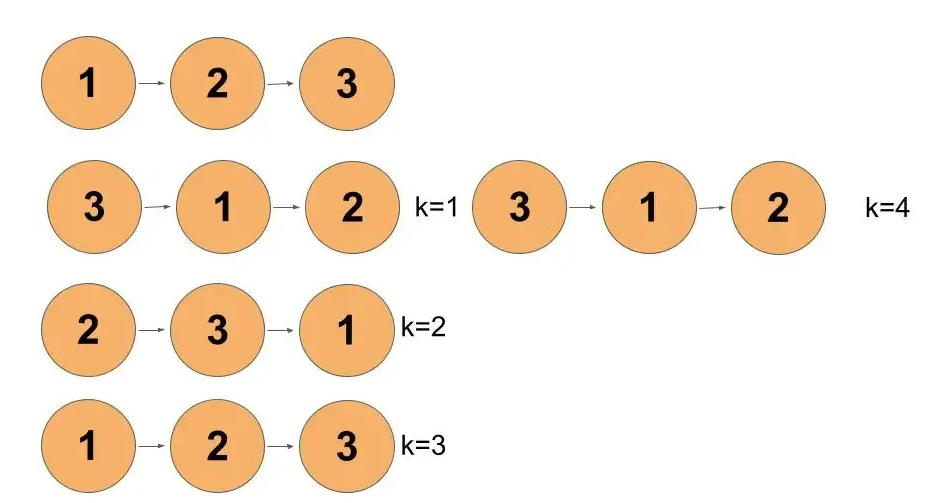Problem Statement: Given the head of a linked list, rotate the list to the right by k places.

Examples:

```Example 1:
Input:
k = 2
Output:
Explanation:
We have to rotate the list to the right twice.```
```Example 2:
Input:
k = 4
Output:
Explanation:```

Solution: Brute Force

Approach:

We have to move the last element to first for each k.

For each k, find the last element from the list. Move it to the first.

Code:

## C++ Code

``````#include<bits/stdc++.h>
using namespace std;

class node {
public:
int num;
node* next;
node(int a) {
num = a;
next = NULL;
}
};
//utility function to insert node at the end of the list
node* newNode = new node(val);
return;
}
while(temp->next != NULL) temp = temp->next;

temp->next = newNode;
return;
}
//utility function to rotate list by k times
for(int i=0;i<k;i++) {
while(temp->next->next != NULL) temp = temp->next;
node* end = temp->next;
temp->next = NULL;
}
}
//utility function to print list
}
return;
}

int main() {
//inserting Node

cout<<"Original list: ";

int k = 2;
the nodes by k times

cout<<"After "<<k<<" iterations: ";
return 0;
}
``````

Output:

Original list: 1->2->3->4->5
After 2 iterations: 4->5->1->2->3

Time Complexity: O(Number of nodes present in the list*k)

Reason: For k times, we are iterating through the entire list to get the last element and move it to first.

Space Complexity: O(1)

Reason: No extra data structures is used for computations

## Java Code

``````import java.util.*;
class Node {
int num;
Node next;
Node(int a) {
num = a;
next = null;
}
}
class TUF {
//utility function to insert node at the end of the list
static Node insertNode(Node head, int val) {
Node newNode = new Node(val);
}
while (temp.next != null) temp = temp.next;

temp.next = newNode;
}
//utility function to rotate list by k times
static Node rotateRight(Node head, int k) {
for (int i = 0; i < k; i++) {
while (temp.next.next != null) temp = temp.next;
Node end = temp.next;
temp.next = null;
}
}
//utility function to print list
}

}

public static void main(String args[]) {
//inserting Node

System.out.println("Original list: ");

int k = 2;
right of the nodes by k times

System.out.println("After " + k + " iterations: ");

}
}``````

Output:

Original list:
1->2->3->4->5
After 2 iterations:
4->5->1->2->3

Time Complexity: O(Number of nodes present in the list*k)

Reason: For k times, we are iterating through the entire list to get the last element and move it to first.

Space Complexity: O(1)

Reason: No extra data structures are used for computations

## Python Code

``````class Node:
def __init__(self, val):
self.val = val
self.next = None

# utility function to insert node at the end of the linked list
newNode = Node(val)
while temp.next != None:
temp = temp.next
temp.next = newNode

# utility function to rotate list by k times
for i in range(k):
while temp.next.next != None:
temp = temp.next
end = temp.next
temp.next = None

# utility function to print list
return

if __name__ == '__main__':
# inserting Node

print("Original list: ", end='')

k = 2
# calling function for rotating right of the nodes by k times

print("After", k, "iterations: ", end='')
printList(newHead)  # list after rotating nodes
``````

Output:

Original list: 1->2->3->4->5
After 2 iterations: 4->5->1->2->3

Time Complexity: O(Number of nodes present in the list*k)

Reason: For k times, we are iterating through the entire list to get the last element and move it to first.

Space Complexity: O(1)

Reason: No extra data structures is used for computations

Solution: Optimal Solution

Approach:

Let’s take an example.

head = [1,2,3,4,5] k = 2000000000

If we see a brute force approach, it will take O(5*2000000000) which is not a good time complexity when we can optimize it.

We can see that for every k which is multiple of the length of the list, we get back the original list. Try to operate brute force on any linked list for k as a multiple of the length of the list.

This gives us a hint that for k greater than the length of the list, we have to rotate the list for k%length of the list. This reduces our time complexity.

Steps to the algorithm:-

• Calculate the length of the list.
• Connect the last node to the first node, converting it to a circular linked list.
• Iterate to cut the link of the last node and start a node of k%length of the list rotated list.

Dry Run:

Let’s calculate the length of the list by iterating on it until it reaches null and increasing the count. Once the length is calculated we will connect the last node to the first node.

Now, the length of the list is 5 and k is 2. k is less than the length of the given list. So, we will have the head of the rotating list at the kth element from the end remove the link from the length-k node from its next node and make it NULL.

Thus, we received our desired output.

Code:

## C++ Code

``````#include<bits/stdc++.h>
using namespace std;

class node {
public:
int num;
node* next;
node(int a) {
num = a;
next = NULL;
}
};
//utility function to insert node at the end of the list
node* newNode = new node(val);
return;
}
while(temp->next != NULL) temp = temp->next;

temp->next = newNode;
return;
}
//utility function to rotate list by k times
//calculating length
int length = 1;
while(temp->next != NULL) {
++length;
temp = temp->next;
}
//link last node to first node
k = k%length; //when k is more than length of list
int end = length-k; //to get end of the list
while(end--) temp = temp->next;
//breaking last node link and pointing to NULL
temp->next = NULL;

}

//utility function to print list
}
return;
}

int main() {
//inserting Node

cout<<"Original list: ";

int k = 2;
node* newHead = rotateRight(head,k);//calling function for rotating right of the nodes by k times

cout<<"After "<<k<<" iterations: ";
return 0;
}
``````

Output:

Original list: 1->2->3->4->5
After 2 iterations: 4->5->1->2->3

Time Complexity: O(length of list) + O(length of list – (length of list%k))

Reason: O(length of the list) for calculating the length of the list. O(length of the list – (length of list%k)) for breaking link.

Space Complexity: O(1)

Reason: No extra data structure is used for computation.

## Java Code

``````import java.util.*;
class Node {
int num;
Node next;
Node(int a) {
num = a;
next = null;
}
}
class TUF{
//utility function to insert node at the end of the list
static Node insertNode(Node head,int val) {
Node newNode = new Node(val);
}
while(temp.next != null) temp = temp.next;

temp.next = newNode;
}
//utility function to rotate list by k times
static Node rotateRight(Node head,int k) {
//calculating length
int length = 1;
while(temp.next != null) {
++length;
temp = temp.next;
}
//link last node to first node
k = k%length; //when k is more than length of list
int end = length-k; //to get end of the list
while(end--!=0) temp = temp.next;
//breaking last node link and pointing to NULL
temp.next = null;

}

//utility function to print list
}

}

public static void main(String args[]) {
//inserting Node

System.out.println("Original list: ");

int k = 2;
of the nodes by k times

System.out.println("After "+k+" iterations: ");

}
}``````

Output:

Original list:
1->2->3->4->5
After 2 iterations:
4->5->1->2->3

Time Complexity: O(length of list) + O(length of list – (length of list%k))

Reason: O(length of the list) for calculating the length of the list. O(length of the list – (length of list%k)) for breaking link.

Space Complexity: O(1)

Reason: No extra data structure is used for computation.

## Python Code

``````class Node:
def __init__(self, val):
self.val = val
self.next = None

# utility function to insert node at the end of the linked list
newNode = Node(val)
while temp.next != None:
temp = temp.next
temp.next = newNode

# utility function to rotate list by k times
if head == None or head.next == None or k == 0:
# calculating length
length = 1
while temp.next != None:
length += 1
temp = temp.next
# link last node to first node
k = k % length  # when k is more than length of list
end = length - k  # to get end of the list
while end:
temp = temp.next
end -= 1
# breaking last node link and pointing to NULL
temp.next = None

# utility function to print list
return

if __name__ == '__main__':
# inserting Node

print("Original list: ", end='')

k = 2
# calling function for rotating right of the nodes by k times

print("After", k, "iterations: ", end='')
printList(newHead)  # list after rotating nodes``````

Output:

Original list: 1->2->3->4->5
After 2 iterations: 4->5->1->2->3

Time Complexity: O(length of list) + O(length of list – (length of list%k))

Reason: O(length of the list) for calculating the length of the list. O(length of the list – (length of list%k)) for breaking link.

Space Complexity: O(1)

Reason: No extra data structure is used for computation.